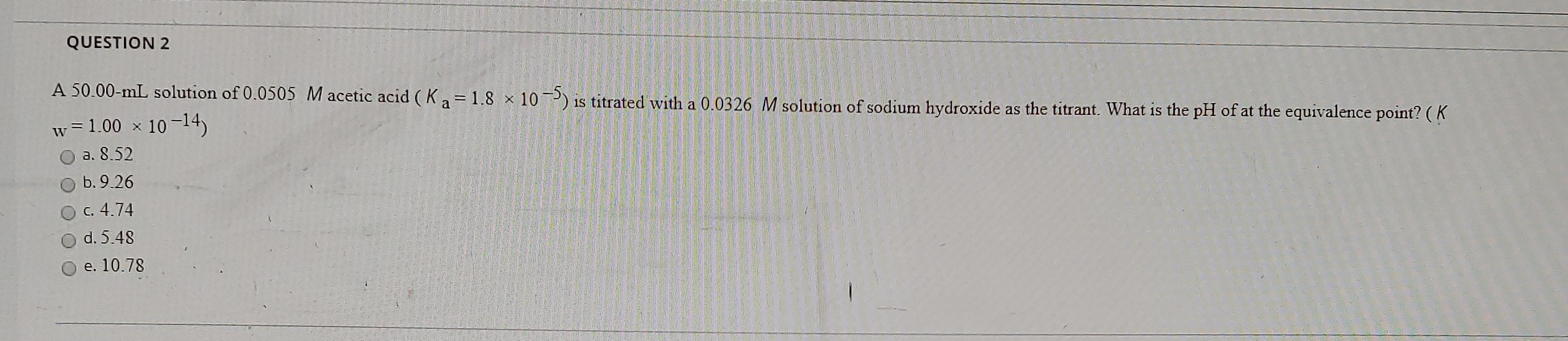# A 50.00 mL solution 0.0505 M acetic acid (Ka = 1.8 x 10^-5) is titrated with a 0.0326 M solution of sodium hydroxide as the titrant. What is the pH of at the equivalence point? (Kw = 1.00 x 10^-14). a. 8.52 b. 9.26 c. 4.74 d. 5.48 e. 10.78